# PHP Operators

Operators in PHP, are simply used to perform operations on variables and values in PHP script.

## PHP 5 Operators

There are following types of operators available in PHP:

• Arithmetic Operators
• Assignment Operators
• Conditional (Ternary) Operators
• Comparison Operators
• Logical Operators
• Increment/Decrement Operators
• String Operator (. and =)
• ?? Operator or Coalescing Operator
• <=> Operator or Spaceship Operator(?:)

## PHP Arithmetic Operators

PHP arithmetic operators are used to perform arithmetical operations, like addition and subtraction etc. Here the following table lists the arithmetic operators available in PHP:

Operator Works
+ This operators adds the two operands
- This operator subtracts the second operand from the first operand
/ This operator divides the numerator by denominator
* This operator multiplies both the operands
% This is a modulus operator, used to find the remainder of an integer division
++ This is an increment operator, increases the value of the integer by one
-- This ia a decrement operator, decreases the value of the integer by one

### Example

Here is an example, uses arithmetic operators in PHP:

```<!DOCTYPE html>
<html>
<body>

<?php
\$num1 = 30;
\$num2 = 50;

\$res = \$num1 + \$num2;
\$res = \$num1 - \$num2;
echo "Subtraction : \$res <br/>";
\$res = \$num1 * \$num2;
echo "Multiplication : \$res <br/>";
\$res = \$num1 / \$num2;
echo "Division : \$res <br/>";
\$res = \$num1 % \$num2;
echo "Modulus : \$res <br/>";
\$res = \$num1++;
echo "Increment : \$res <br/>";
\$res = \$num1--;
echo "Decrement : \$res <br/>";
?>

</body>
</html>```

Here is the output produced by the above PHP script: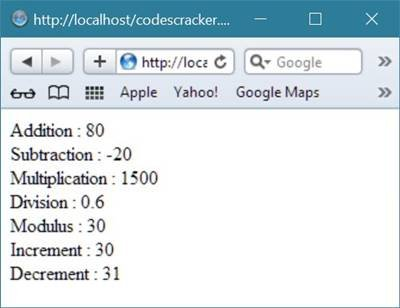## PHP Assignment Operators

The assignment operators in PHP, are used with numeric values to write the value to a variable. And the basic arithmetic operator in PHP (which is "="), is used to assign the value of the right to the left operand. Here, the following table lists the assignment operators available in PHP:

Operator Name Works
= Simple Assignment Operator This operator assigns the values from the right side operands to the left side operand
+= Add and Assignment Operator This operator is used to add the right operand to the left operand and then assign the result to the left operand
-= Subtract and Assignment Operator This operator subtracts the right operand from the left operand and then assign the result to the left operand
/= Divide and Assignment Operator This operator divides the left operand with the right operand and then assign the result to the left operand
*= Multiply and Assignment Operator This operator multiplies the right operand with the left operand and then assign the result to the left operand
%= Modulus and Assignment Operator This operator takes the modulus using both the operands and then assign the result to the left operand

### Example

Here is an example, uses assignment operators available in PHP:

```<!DOCTYPE html>
<html>
<body>

<?php
\$num1 = 42;
\$num2 = 20;

\$res = \$num1 + \$num2;
echo "Addition Operation Result: \$res <br/>";
\$res += \$num1;
echo "Add AND Assignment Operation Result: \$res <br/>";
\$res -= \$num1;
echo "Subtract AND Assignment Operation Result: \$res <br/>";
\$res *= \$num1;
echo "Multiply AND Assignment Operation Result: \$res <br/>";
\$res /= \$num1;
echo "Division AND Assignment Operation Result: \$res <br/>";
\$res %= \$num1;
echo "Modulus AND Assignment Operation Result: \$res <br/>";
?>

</body>
</html>```

It will display the following result in the browser: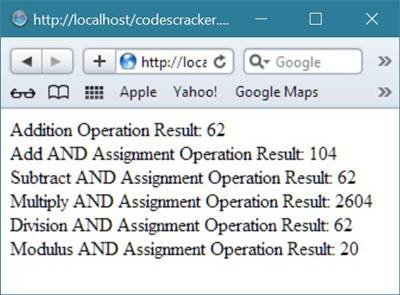Now let's take an example of PHP basic assignment operator.

```<html>
<title>PHP Basic Assignment (=) Operator</title>
<body>
<?php
\$fname = "Codes";
\$lname = (\$name = "Cracker");
echo \$fname;
echo "<br/>";
echo \$lname;
echo "<br/>";
echo \$name;
echo "<br/>";
\$fullname = (\$flname = \$fname.\$lname);
echo \$fullname;
echo "<br/>";
echo \$flname;
?>
</body>
</html>```

Here is the sample output produced by the above basic assignment operator in PHP: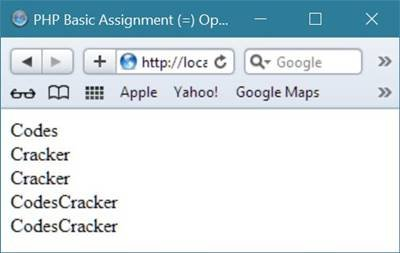## PHP Comparison Operators

PHP comparison operators are used to compare two values. Here the following table lists the comparison operators available in PHP:

Operator Name Operation Result When
== Equal \$num1 == \$num2 Returns true if \$num1 is equal to \$num2
=== Identical \$num1 === \$num2 Returns true If \$num1 is equal to \$num2, and they are of the same type
!= Not equal \$num1 != \$num2 Returns true If \$num1 is not equal to \$num2
!== Not identical \$num1 !== \$num2 Returns true If \$num1 is not equal to \$num2, or they aren't of the same type
< Less than \$num1 < \$num2 Returns true If \$num1 is less than \$num2
> Greater than \$num1 > \$num2 Returns true If \$num1 is greater than \$num2
<> Not equal \$num1 <> \$num2 Returns true If \$num1 isn't equal to \$num2
<= Less than or equal to \$num1 <= \$num2 Returns true If \$num1 is less than or equal to \$num2
<= Greater than or equal to \$num1 <= \$num2 Returns true If \$num1 is greater than or equal to \$num2

### Example

Here is an example, uses comparison operators available in PHP:

```<!DOCTYPE html>
<html>
<body>

<?php
\$num1 = 42;
\$num2 = 20;

if(\$num1 == \$num2)
{
echo "Check1 : num1 is equal to num2<br/>";
}
else
{
echo "Check1 : num1 is not equal to num2<br/>";
}
if(\$num1 > \$num2)
{
echo "Check2 : num1 is greater than  num2<br/>";
}
else
{
echo "Check2 : num1 is not greater than num2<br/>";
}
if(\$num1 < \$num2)
{
echo "Check3 : num1 is less than  num2<br/>";
}
else
{
echo "Check3 : num1 is not less than num2<br/>";
}
if(\$num1 != \$num2)
{
echo "Check4 : num1 is not equal to num2<br/>";
}
else
{
echo "Check4 : num1 is equal to num2<br/>";
}
if(\$num1 >= \$num2)
{
echo "Check5 : num1 is either grater than or equal to num2<br/>";
}
else
{
echo "Check5 : num1 is neither greater than nor equal to num2<br/>";
}
if(\$num1 <= \$num2)
{
echo "Check6 : num1 is either less than or equal to num2<br/>";
}
else
{
echo "Check6 : num1 is neither less than nor equal to num2<br/>";
}
?>

</body>
</html>```

Here is the output produced by this PHP script: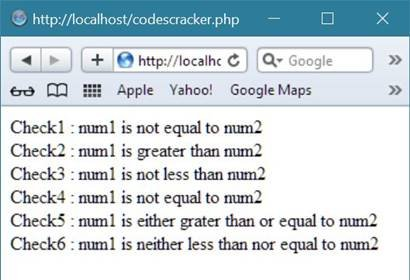## PHP Increment/Decrement Operators

PHP increment/decrement operators are used to increment/decrement value of the variable. Here, the following table lists the increment/decrement operators available in PHP:

Operator Name Meaning
\$num1++ Post-increment Returns \$num1, then increments \$num1 by one
++\$num1 Pre-increment Increments \$num1 by one, then returns \$num1
\$num1-- Post-decrement Returns \$num1, then decrements \$num1 by one
--\$num1 Pre-decrement Decrements \$num1 by one, then returns \$num1

Simply you can say that that PHP incrementing (++) Operator is used to increment the value of a PHP variable by 1.

You can set incrementing operator before or after the variable.

PHP decrementing (--) operator is used to decrement the value of a PHP variable by 1.

You can set decrementing operator before or after the variable.

### Example

Here is an example, uses increment/decrement operators available in PHP:

```<!DOCTYPE html>
<html>
<body>

<?php
\$num1 = 0;
\$num2 = 0;

echo "\$num1"."<br/>";

\$num2 = ++\$num1;
echo "\$num2"."<br/>";

\$num2 = \$num1++;
echo "\$num2"."<br/>";

\$num2 = --\$num1;
echo "\$num2"."<br/>";

\$num2 = \$num1--;
echo "\$num2"."<br/>";
?>

</body>
</html>```

The above PHP script produce the following output: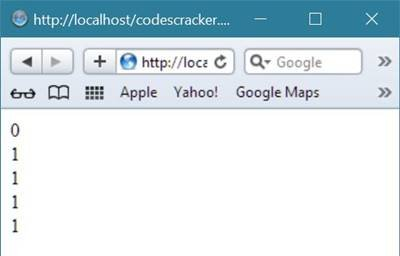Let's take another example of increment(++) and decrement(--) operator in PHP.

```<html>
<title>PHP increment and decrement operator</title>
<body>
<?php
# PHP increment (++) and decrement (--) operator
# example with all the four option, that is,
# pre-increment, post-increment,
# pre-decrement and post-decrement
\$value1 = 5;
\$value2 = 5;
\$value3 = \$value1++;
\$value4 = ++\$value2;
echo \$value1;
echo "<br/>";
echo \$value2;
echo "<br/>";
echo \$value3;
echo "<br/>";
echo \$value4;
echo "<hr/>";

\$valueb1 = 5;
\$valueb2 = 5;
\$valueb3 = \$valueb1--;
\$valueb4 = --\$valueb2;
echo \$valueb1;
echo "<br/>";
echo \$valueb2;
echo "<br/>";
echo \$valueb3;
echo "<br/>";
echo \$valueb4;
echo "<hr/>";
?>
</body>
</html>```

Here is the sample output produced by the above PHP increment and decrement example code: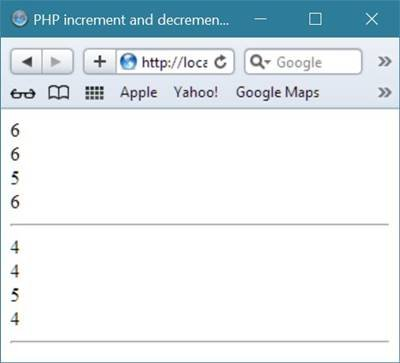## PHP Logical Operators

PHP logical operators are used to combile conditional statements. Here, the following table lists the logical operators available in PHP:

Operator Name Operation Result When
or Or \$num1 or \$num2 True If either \$num1 or \$num2 is true
and And \$num1 and \$num2 True If both \$num1 and \$num2 are true
xor Xor \$num1 xor \$num2 True If either \$num1 or \$num2 is true, but not both
|| Or \$num1 || \$num2 True If either \$num1 or \$num2 is true
&& And \$num1 && \$num2 True If both \$num1 and \$num2 are true
! Not !\$num1 True If \$num1 is not true

### Example

Here is an example, uses logical operators available in PHP:

```<!DOCTYPE html>
<html>
<body>

<?php
\$num1 = 42;
\$num2 = 0;

if(\$num1 && \$num2)
{
echo "Check1 : Both num1 and num2 are true<br/>";
}
else
{
echo "Check1 : Either num1 or num2 is false<br/>";
}
if(\$num1 and \$num2)
{
echo "Check2 : Both num1 and num2 are true<br/>";
}
else
{
echo "Check2 : Either num1 or num2 is false<br/>";
}
if(\$num1 || \$num2)
{
echo "Check3 : Either num1 or num2 is true<br/>";
}
else
{
echo "Check3 : Both num1 and num2 are false<br/>";
}
if(\$num1 or \$num2)
{
echo "Check4 : Either num1 or num2 is true<br/>";
}
else
{
echo "Check4 : Both num1 and num2 are false<br/>";
}

\$num1 = 10;
\$num2 = 20;

if(\$num1)
{
echo "Check5 : num1 is true <br/>";
}
else
{
echo "Check5 : num1  is false<br/>";
}
if(\$num2)
{
echo "Check6 : num2 is true <br/>";
}
else
{
echo "Check6 : num2  is false<br/>";
}
if(!\$num1)
{
echo "Check7 : num1 is true <br/>";
}
else
{
echo "Check7 : num1  is false<br/>";
}
if(!\$num2)
{
echo "Check8 : num2 is true <br/>";
}
else
{
echo "Check8 : num2  is false<br/>";
}
?>

</body>
</html>```

Here is the output produced by the above PHP script: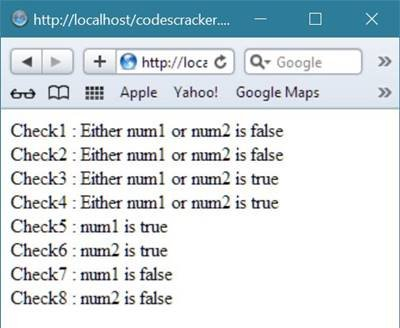Let's take an example of logical AND and logical OR operator in PHP.

```<html>
<title>PHP Logical AND (&&) and OR (||) Operator</title>
<body>

<?php
\$num1 = 5;
\$num2 = 10;
\$num3 = 5;
\$num4 = 10;
if(\$num1==\$num2 && \$num1==\$num3)
{
echo "1. Both expression returns to be true.<br/>";
}
if(\$num1==\$num2 || \$num1==\$num3)
{
echo "2. Either of the two expression returns to be true.</br>";
}
if(\$num1==\$num3 && \$num2==\$num4)
{
echo "3. Both expression returns to be true.<br/>";
}
if(\$num1==\$num3 || \$num2==\$num4)
{
echo "4. Either of the two expression returns to be true.<br/>";
}
?>
</body>
</html>```

Here is the sample output of the above logical AND and logical OR operator in PHP:## PHP Conditional Operator

PHP conditional operator is an important operator available in any programming language. The conditional operator is just evaluates like if-else operator. Here is the general form of the conditional operator in PHP:

`var = expression ? value1 : value2;`

Here, expression is any valid expression in PHP. If the expression evaluates to true, then value1 is assigned to the variable (var), otherwise, value2 is assigned to the variable (var). Here is an example, uses conditional operator in PHP:

```<!DOCTYPE html>
<html>
<body>

<?php
\$num1 = 10;
\$num2 = 20;

\$result = (\$num1 > \$num2 ) ? \$num1 : \$num2;
echo "Check1 : Value of result is \$result<br/>";

\$result = (\$num1 < \$num2 ) ? \$num1 : \$num2;
echo "Check2 : Value of result is \$result<br/>";
?>

</body>
</html>```

It will display the following result: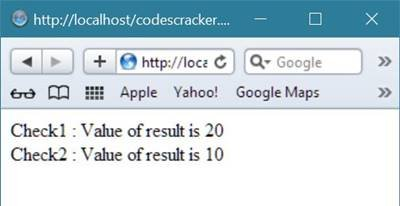## PHP Ternary Operator

PHP ternary operator can also be called as PHP conditional operator.

PHP ternary operator or ?: operator in PHP is something similar to the PHP if statement, as in ?: operator if the condition return to be true, then the value of the first block will be returned otherwise, value of the second block will be returned.

Here is the general form of the PHP ternary operator (?:)

`\$val = expression ? firstValue : secondValue`

Let's take an example demonstrating ternary operator in PHP.

```<html>
<title>PHP ternary operator (?:)</title>
<body>

<?php
\$num1 = 5;
\$num2 = 10;
\$val = \$num1>\$num2 ? "right" : "wrong";
echo \$val;
echo "<hr/>";

\$num1 = 5;
\$num2 = 10;
\$val = \$num1<\$num2 ? "right" : "wrong";
echo \$val;
echo "<hr/>";

\$num1 = 5;
\$num2 = 10;
\$val = \$num1>\$num2 ? 1 : 0;
echo \$val;
echo "<hr/>";

\$num1 = 5;
\$num2 = 10;
\$val = \$num1<\$num2 ? 1 : 0;
echo \$val;
echo "<hr/>";
?>
</body>
</html>```

Below is the sample output produced by the above PHP ternary operator example code: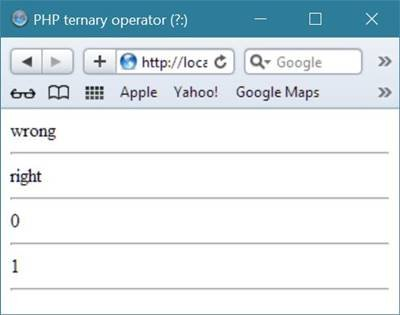## PHP String Operator (. and =)

The PHP string operator, that is, dot (.) is used to combine any two string in PHP and .= is used to combine two variable and assign it into a variable.

Let's take an example demonstrating the string operator in PHP

```<html>
<title>PHP String Operator (. and =)</title>
<body>
<?php
\$fname = "Codes";
\$lname = "Cracker";
\$fullname = \$fname . \$lname;
echo \$fullname;
echo "<hr/>";
\$firstname = "Codes";
\$firstname .= "Cracker";
echo \$firstname;
echo "<hr/>";
?>
</body>
</html>```

Here is the sample output produced by the above PHP string operator example code:## PHP <=> or Spaceship Operator

The PHP <=> operator or spaceship operator is a new operator in PHP, came in PHP 7th version, that is, PHP 7.

The <=> operator in PHP is used to compare expressions.

The spaceship or <=> operator in PHP will return the following three values in three different situations:

• return -1, if the first expression is less than second expression
• return 0, if the first expression is equal to second expression
• return 1, if the first expression is greater than second expression

Let's take an example illustrating the spaceship operator in PHP.

```<html>
<title>PHP <=> Operator or Spaceship Operator</title>
<body>
<?php
print (5 <=> 5);
echo "<br/>";
print (3 <=> 5);
echo "<br/>";
print (5 <=>3);
echo "<hr/>";
print ("C" <=> "C");
echo "<br/>";
print ("A" <=> "C");
echo "<br/>";
print ("C" <=> "A");
echo "<hr/>";
?>
</body>
</html>```

The above PHP spaceship operator example code will produce the following output: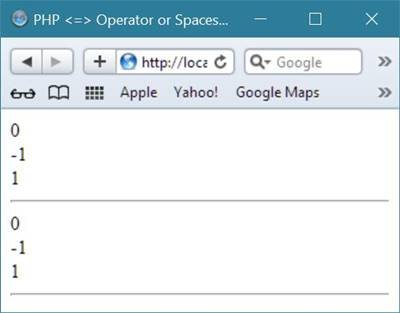## PHP ?? or Coalescing Operator

The ?? operator in PHP is introduced in its 7th version, that is, PHP 7.

In PHP ?? or Coalescing operator, if the first operand of this operator is set then it returns its first operand, otherwise second operand will be returned by this operator.

Here is an example that demonstrates PHP Coalescing or ?? operator.

```<html>
<title>PHP ?? Operator or Coalescing Operator</title>
<body>
<?php
\$firstname = "Codes";
\$lastname = "Cracker";
\$fullname = \$_POST['name'] ?? 'name is empty';
echo \$fullname;
echo "<hr/>";
\$name = \$firstname ?? "firstname is not initialized";
echo \$name;
echo "<hr/>";
\$name = \$surname ?? "surname is undefined";
echo \$name;
echo "<hr/>";
\$name = \$fullname ?? "fullname is set previously";
echo \$fullname;
?>
</body>
</html>```

Here is the sample output of the above PHP Coalescing operator.## PHP Operators Precedence

Associativity Operator
left -> ::
clone new
[
**
right ++ -- ~ (int) (float) (string) (array) (object) (bool) @
instanceof
!
left * / %
left + - .
left << >>
< <= > >=
== != === !== <> <=>
&
^
|
&&
||
??
? :
right = += -= *= **= /= .= %= &=
and
xor
or

Tools
Calculator